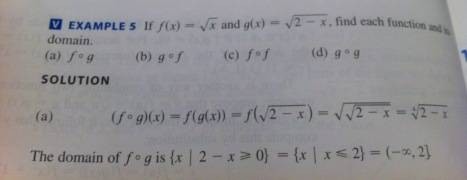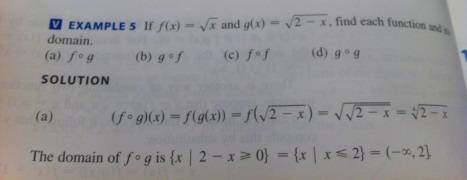# F of G function question - Square root inside a square root?

nukeman

## Homework Statement

The image has the question I don't quite understand!## The Attempt at a Solution

I understand how to get √(√2 - x) but I don't get how they end up with: 4√2 - x

?

Last edited:

Mentor

## Homework Statement

The image has the question I don't quite understand!## The Attempt at a Solution

I understand how to get √(√2 - x) but I don't get how they end up with: 4√2 - x
Your notation is incorrect. This is what you wrote:
$$\sqrt{\sqrt{2} - x}$$

What you should have written is
$$\sqrt{\sqrt{2-x}}$$

Without using LaTeX, as I did, you could have written √(√(2 - x))

Do you see the difference?

nukeman
Your notation is incorrect. This is what you wrote:
$$\sqrt{\sqrt{2} - x}$$

What you should have written is
$$\sqrt{\sqrt{2-x}}$$

Without using LaTeX, as I did, you could have written √(√(2 - x))

Do you see the difference?

Ah yea, I see the difference. Gotta start writing in latex!

I still don't understand the problem as I pointed out in the picture. ?

Mentor
Write the expression in exponent form rather than radical form.